# Reversing The row Order Of Values In A List in Excel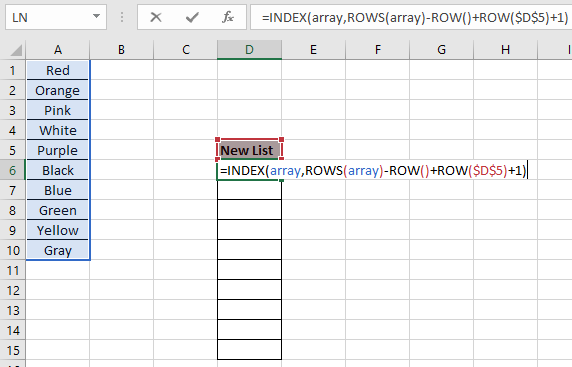In this article, we will learn How to use the ... function in Excel.

Scenario :

In Excel, you can sort values in ascending or descending. But there is no default way by excel to reverse or flip a list of values. So we couldn't sit like this, we can perform the task using given excel formulas. Let's understand the formula as explained below.

OFFSET - COUNTA formula to reverse list of values

For this we will use a combination of three functions and get to know a little about them. The below formula returns the last value from the range in (A2:A7).

formula:

 =OFFSET(\$A\$2, COUNTA(array)-ROW()+1,0)

array : list of values starting with A2 cell

\$A\$2 : first array cell reference

INDEX - ROWS formula to reverse list of values

Now we will construct a formula using the above explained function and a simple logic. Firstly, we need to get the last value of the list into the first value of the new reverse list. Then we need to carry on the formula to other cells using a simple logic. Use the formula in the D6 cell.

Formula in D6 cell:

 = INDEX ( array , ROWS (array) - ROW () + ROW (\$D\$5) +1)

array : list of values starting

\$D\$5 : one cell before the first output cell. (D6 - 1 = D5)

Example :

All of these might be confusing to understand. Let's understand how to use the function using an example. Here we have a list of values and a New List column.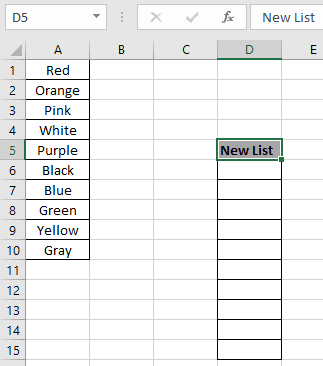We need to fill the New list values with reverse order of the list values. Array is taken as named range for the A1:A10 array.

Use the formula in the D6 cell:

 = INDEX ( array , ROWS ( array ) - ROW( ) + ROW ( \$D\$5 ) +1 )

Explanation:

• ROW ( ) will return 6, the corresponding row number for the cell.
• ROW( \$D\$5 ) the cell_reference for the just previous cell will return 5, the row number for the D5 cell.
• \$ sign with cell reference is used to fix the cell reference as excel extends the cell reference as you extend the use of the formula.
• ROWS(array) will returns the number of values in the array which is 10 in this case.
• Index function then searches the { 10 -6 +5 +1 } th element in the array =  10th element which is the last element in the array.Here values to the function is given as cell reference.

Press Enter to get the result.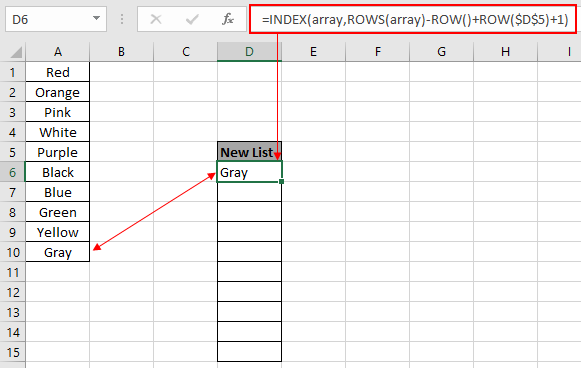As you can see in the above snapshot that the formula returns the last element in the first cell of the New List.

Copy the formula to the rest of the cells using the Ctrl + D shortcut key or drag down option in excel.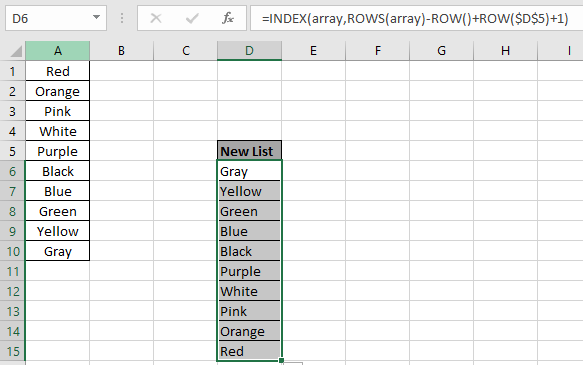As you can see from the above snapshot that the New List column is filled with values as required.

There's an easy method to do the same. But it's just for the sake of time saving and result orienting.

1. Type the number 1 & 2 in the first and second in the adjacent column of the Values.
2. Then select the two cells and drag it down till the Index column fills.
3. Ctrl + Shift + L to apply the filter option in excel.
4. Then reverse the sorting of numbers to Largest to Smallest will reverse sort the Values list too.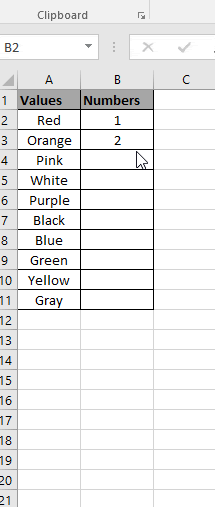As you can see in the gif above that the Values column is in reverse sort order.

One Note: The formula explained above works fine when the required array can be anywhere.

Use the formula when the required values starts from the first row of the worksheet.

 = INDEX ( array , ROWS ( array ) - ROW ( ) + 1 )

OFFSET - COUNTA formula to reverse list of values

For this we will use a combination of three functions and get to know a little about them. The below formula returns the last value from the range in (A2:A7).

formula:

 =OFFSET(\$A\$2,COUNTA(\$A\$2:\$A\$7)-ROW()+1,0)

array : list of values starting with A2 cell

\$A\$2 : first array cell reference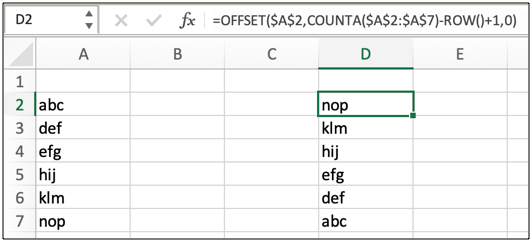Copy and paste the formula using the Ctrl + D or drag the box at the right bottom of the cell. This will create a list of value in reverse order

Here are some observational outputs using the above formula.

Notes:

1. You can use any of the formula to reverse the list of values
2. The formula works fine for both numerical and text values.

Hope this article about Reversing The row Order Of Values In A List in Excel is explanatory. Find more articles on calculating values and related Excel formulas here. If you liked our blogs, share it with your friends on Facebook. And also you can follow us on Twitter and Facebook. We would love to hear from you, do let us know how we can improve, complement or innovate our work and make it better for you. Write to us at info@exceltip.com.

Related Articles :

How to use Shortcut Keys for Sort & filter in Excel : Use Ctrl + Shift + L from keyboard to apply sort and filter on table in Excel.

The SORT Function in Excel 365 (new version) : The SORT function  returns the sorted array by the given column number in the array. It also works on horizontal data.

Sort numbers using Excel SMALL Function : To sort numbers using formula in Excel 2016 and older, you can use the SMALL function with other helping functions.

Sort Numeric Values with Excel RANK Function : To sort the numeric values we can use the Rank function. The formula is

Excel Formula to Sort Text : To sort text values using formula in excel we simply use the COUNTIF function. Here is the formula

Excel Formula to Extract Unique Values From a List : To find all unique values from a list in Excel 2016 and older versions, we use this simple formula.

Popular Articles :

How to use the VLOOKUP Function in Excel : This is one of the most used and popular functions of excel that is used to lookup value from different ranges and sheets.

How to use the IF Function in Excel : The IF statement in Excel checks the condition and returns a specific value if the condition is TRUE or returns another specific value if FALSE.

How to use the SUMIF Function in Excel : This is another dashboard essential function. This helps you sum up values on specific conditions.

How to use the COUNTIF Function in Excel : Count values with conditions using this amazing function. You don't need to filter your data to count specific values. Countif function is essential to prepare your dashboard.

Terms and Conditions of use

The applications/code on this site are distributed as is and without warranties or liability. In no event shall the owner of the copyrights, or the authors of the applications/code be liable for any loss of profit, any problems or any damage resulting from the use or evaluation of the applications/code.